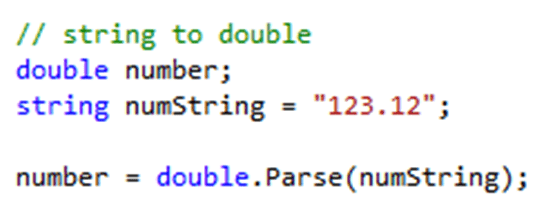# Integral Data Types

### Identifiers and Data Types

Every variable has:
• a space in memory
• an identifier = name
• a data type = the type of data that will be stored inside this location
•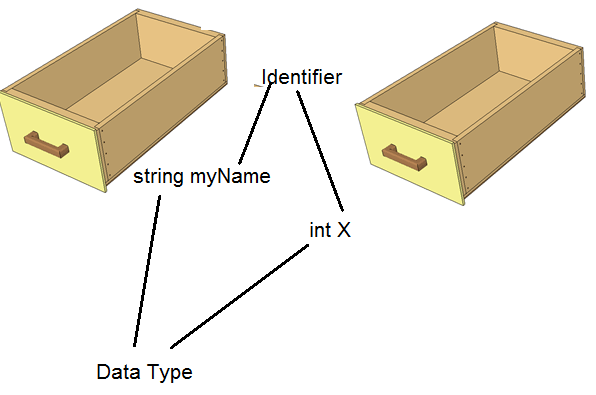• giving a variable a name and a data type is called a declaration
• a declaration reserves storage for the value you will put into the variable
• Example: int myAge;

### Integral data types are as follows:

• Types that store whole numbers
• byte, sbyte, short, ushort, int, uint, long, and ulong
• Variables of type int
• Store (or hold) integers, or whole numbers
• Shorter integer types:
• byte, sbyte (which stands for signed byte), short (short int), or ushort (unsigned short int)

#### Double Data Type

• Data Type for decimal numbers. For example: double decimalNumber; decimalNumber = 14.5;

#### Floating-Point Number

Contains decimal positions and the data types are as follows:
• float: Can hold up to seven significant digits of accuracy
• double: Can hold 15 or 16 significant digits of accuracy
• decimal: Has a greater precision and a smaller range and suitable for financial and monetary calculations

#### Char Data Type

Chars can be assigned values as follows: char letterGrade; letterGrade = 'B' or char letterGrade = 'B' This can be changed later for example; letterGrade = 'A' chars are always enclosed in Single Quotes (').

#### Bool Data Type

bool can be assigned values as follows: bool married; married = true; Or bool married = true; and then change later for example: married = false; More details on C# data types are included here: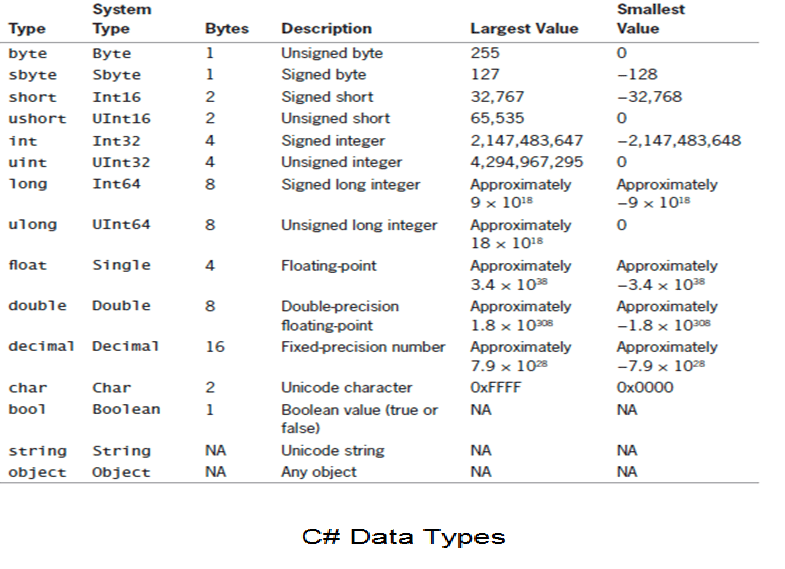### Arithmetic Operators

Operators in C# are shown in this table: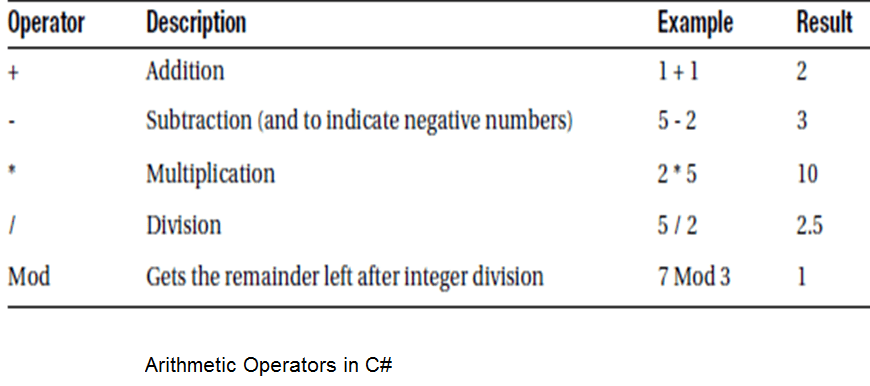Operator Precedence in C# depends on order in which the operators are evaluated:
• First: exponentiation
• Second: multiplication and division
• parentheses () will change the order

Examples: What is the value of number?

1. int number; number = 4 + 2 * 3;
2. int number; number = (4 + 2) * 3
1. number = 10
2. number = 18

### Type Conversion (Casting)

Casting means changing the variable from one data type to another. Casting an integer to a double (implicit casting). See example here: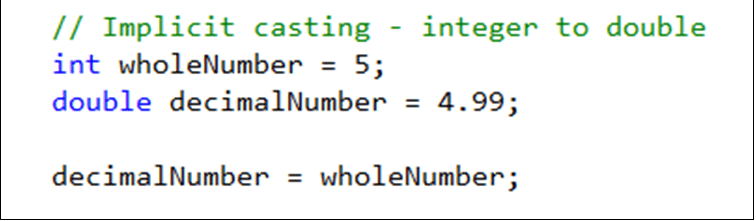Casting a double to an integer (explicit casting) See example: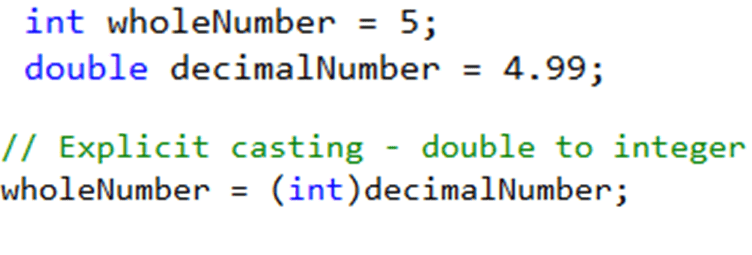Casting an integer to a string. See example: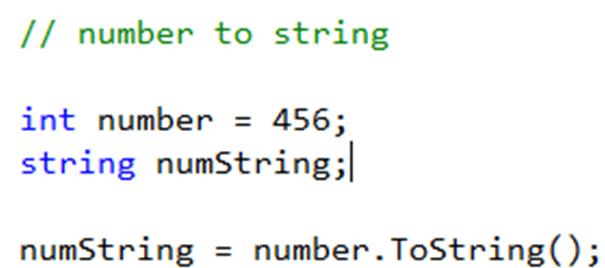Casting an string to a number (int). See example: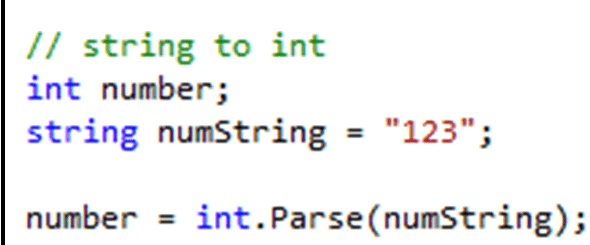Casting an string to a number (double). See example: